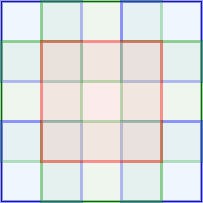Hi,

I am trying to understand how Adaptive Average Pooling 2D works but I could not find a detailed explanation on google.

I did a small test on a 5x5 tensor.

``````import torch
import torch.nn.functional as F

x = torch.rand(1, 1, 5, 5)
# x:  tensor([[[[0.7984, 0.6614, 0.4994, 0.9424, 0.8370],
#               [0.9245, 0.0681, 0.8324, 0.9549, 0.8145],
#               [0.6221, 0.1108, 0.5670, 0.2415, 0.4548],
#               [0.1318, 0.3454, 0.5996, 0.4357, 0.1078],
#               [0.3747, 0.5511, 0.5398, 0.2429, 0.6817]]]])
# ret:  tensor([[[[0.6131, 0.6598, 0.8872],
#                 [0.3671, 0.4617, 0.5015],
#                 [0.3507, 0.4524, 0.3670]]]])
``````

It seems the average is computed in the cells as followsI search the source code of PyTorch and it leads me to SpatialAdaptiveAveragePooling.c and its cuda counterpart. There are two ways to do the partition, defined as

``````#define START_IND(a,b,c) (int)floor((float)(a * c) / b)
#define END_IND(a,b,c) (int)ceil((float)((a + 1) * c) / b)
// #define START_IND(a,b,c) a * c / b
// #define END_IND(a,b,c)  (a + 1) * c / b + ((a + 1) * c % b > 0)?1:0
``````
``````int istartH = START_IND(oh, osizeH, isizeH);
int iendH   = END_IND(oh, osizeH, isizeH);
int kH = iendH - istartH;
``````

In my example, the height and width are same so we only consider height. We have the followings:

``````oh           0     1     2
istartH      0     1     3
iendH        1     3     5
kH           1     2     2
``````

But it seems the actual result computes these statistic following the definition in the comment.

``````// #define START_IND(a,b,c) a * c / b
// #define END_IND(a,b,c)  (a + 1) * c / b + ((a + 1) * c % b > 0)?1:0
``````
``````oh           0     1     2
istartH      0     1     3
iendH        2     4     5
kH           2     3     2
``````

Is there something in the source I missed?

Many thanks!

4 Likes